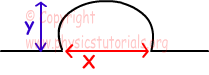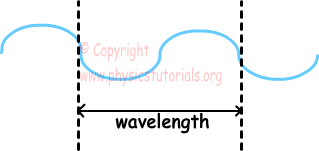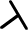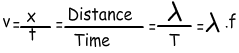Properties of Waves

Properties of Waves

In this section we will learn basic properties of waves. We have learned transverse and longitudinal waves in last section. Now we use them and try to explain basic concepts of wave phenomena as; wavelength, velocity, amplitude, pulse, frequency.

Pulse:  one wave motion created at the spring.Where; x is the pulse length and y is the amplitude (height of the pulse).

Wavelength:It is the distance between two points of two waves having same characteristics.wavelength is shown with the greek letter "" and unit of it is "m".

Period: Time required for production of one wave is called period. It is shown with letter "T" and its unit is "s".

Frequency: It is the number of waves produced in a given unit of time. It is shown with letter "f" and its unit is "1/s".

Periodic Wave: If the wave source produces equal number of waves in equal times, then this wave called periodic wave.

f=1/T

Velocity of the Wave: Velocity of the wave is constant in a given medium. However, if the medium is changed then velocity of the wave is also changed.  We show velocity with "v" and its unit is m/s.Waves Exams and Solutions

Author: